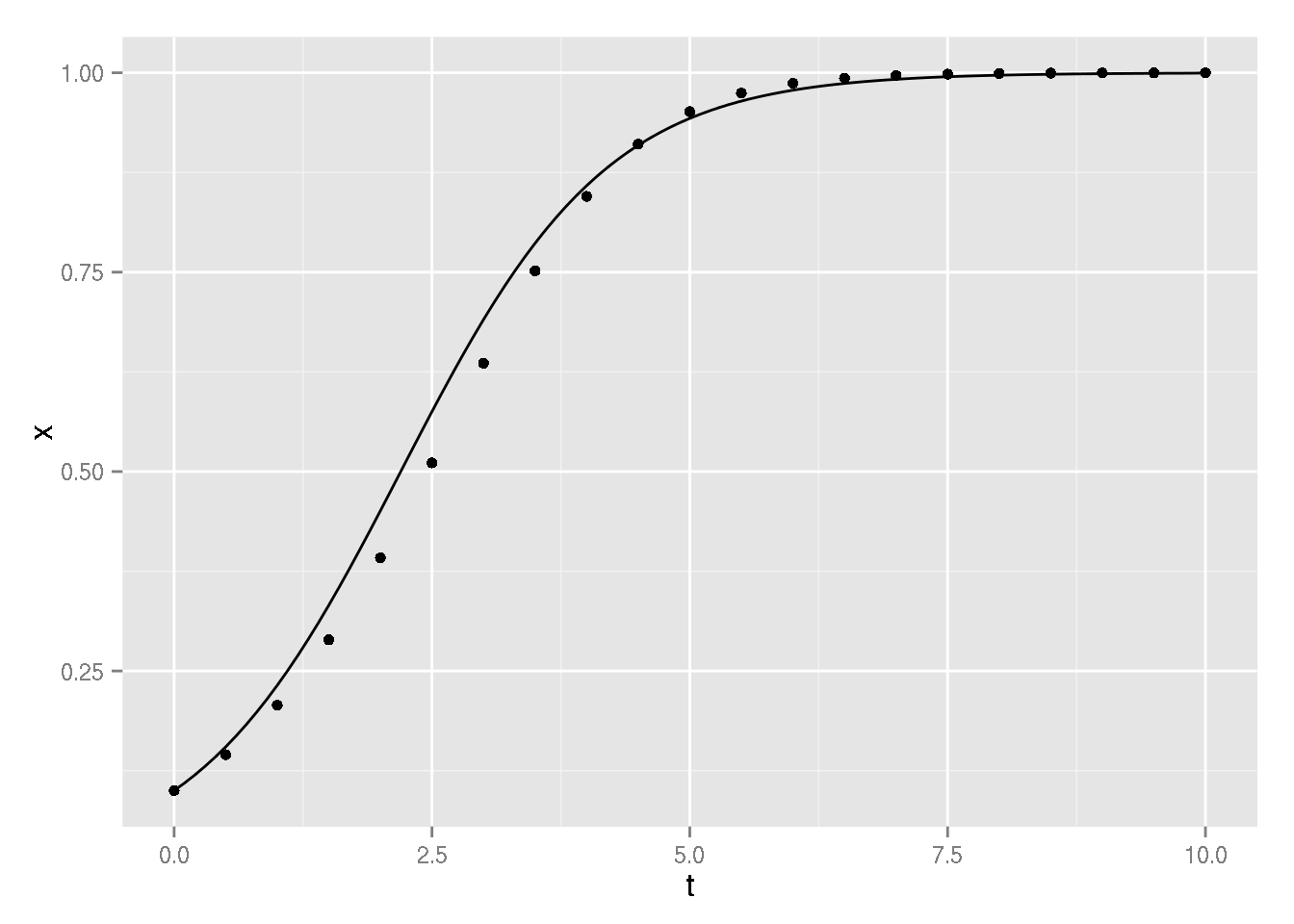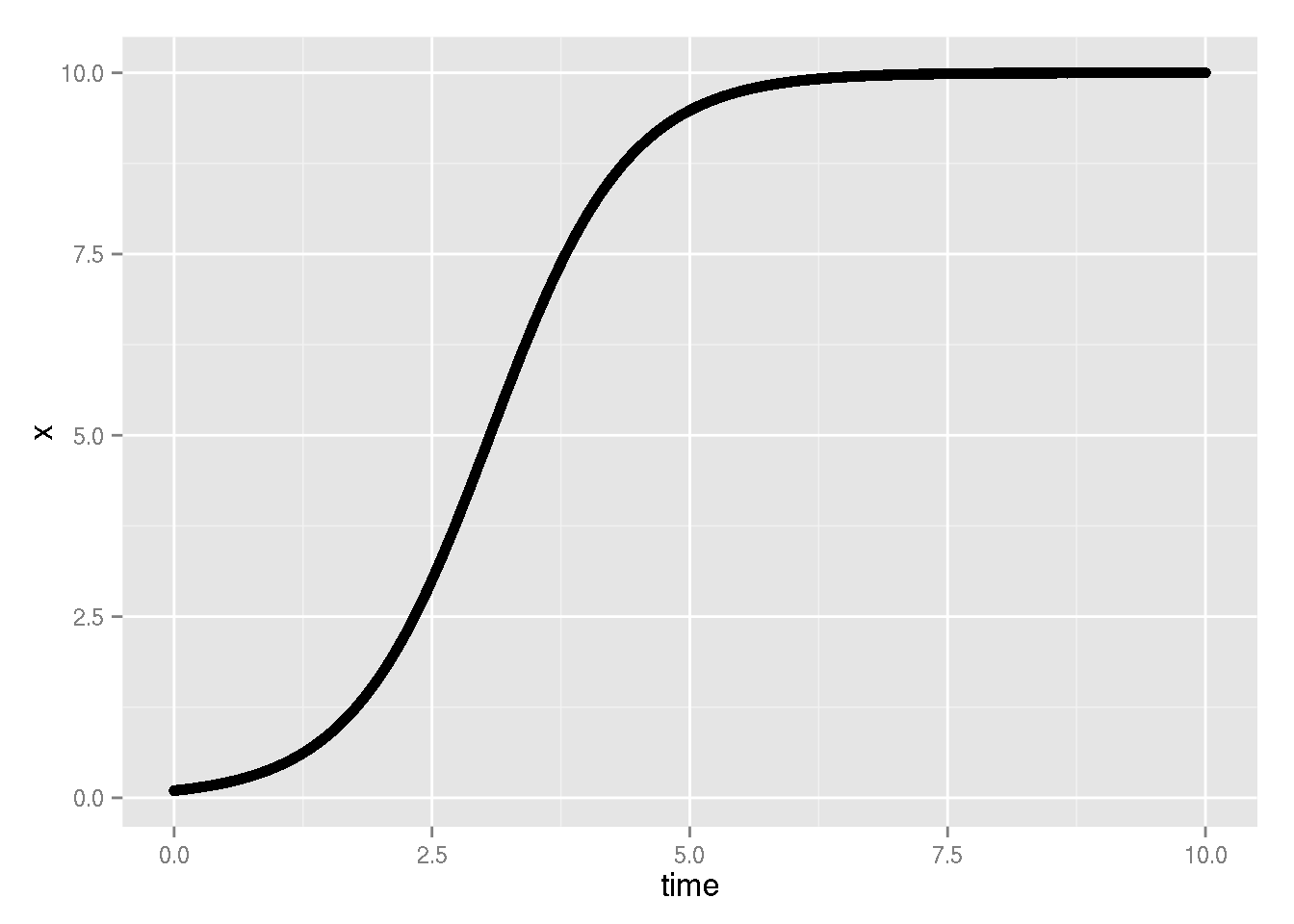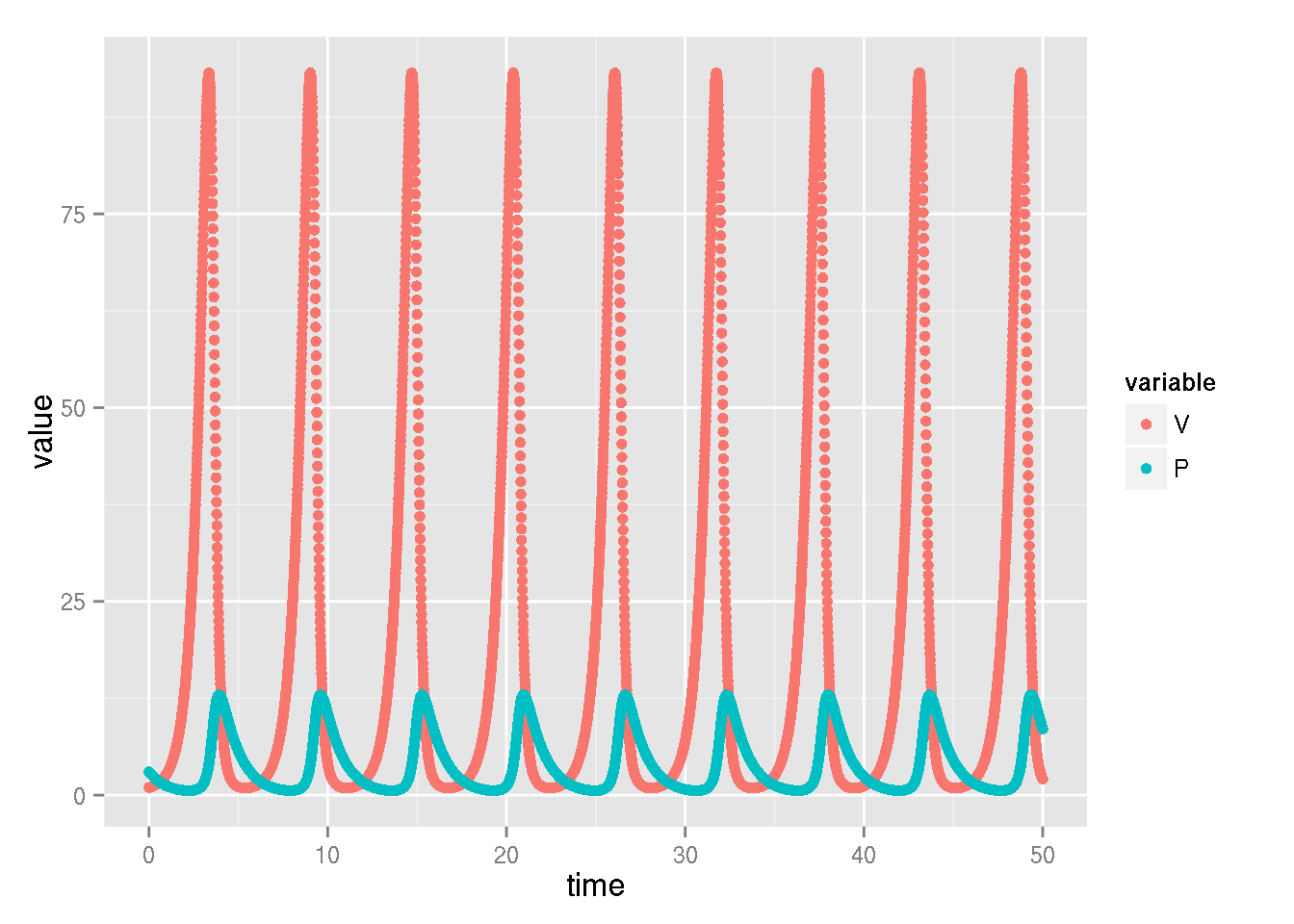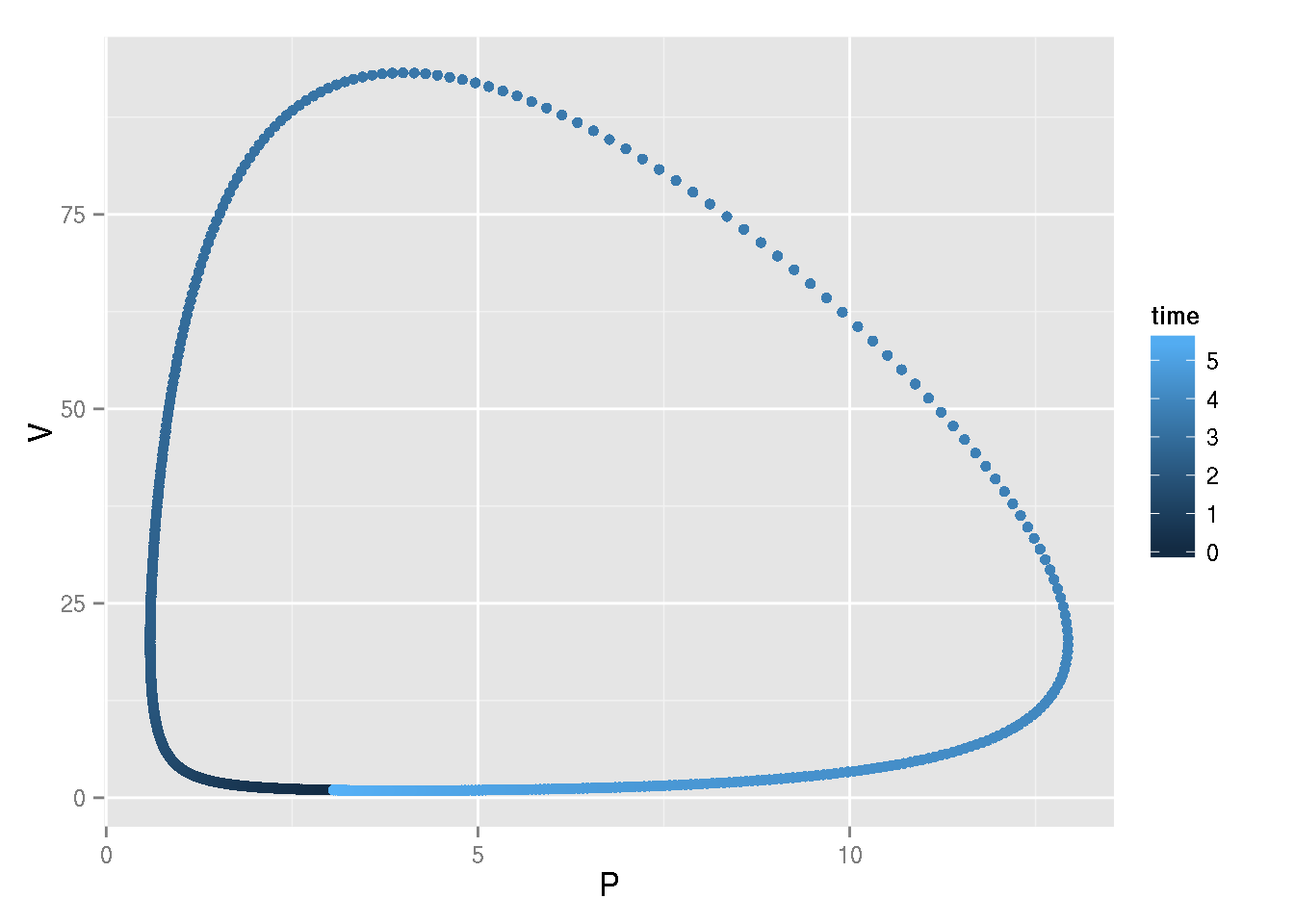# Numerically solving differential equations with R

This is a brief description of what numerical integration is and a practical tutorial on how to do it in R.

## Software required

### R

In order to run the R codes in this document in your own computer, you need to install the following software:

• R, along with the packages from CRAN:
• deSolve, a library for solving differential equations
• ggplot2, a library for plotting
• reshape2, for manipulating data.frames
• rmarkdown, para converter este documento em html ou pdf.
• Opcionalmente, pode-se usar o ambiente RStudio para editar e converter documentos no formato rmarkdown

To install R, download it from its homepage (Windows or Mac): http://www.r-project.org/. On Linux, you can install it using your distribution’s prefered way, e.g.:

• Debian/Ubuntu: sudo apt-get install r-base
• Fedora: sudo yum install R
• Arch: sudo pacman -S r

To install the packages, all you have to do is run the following in the R prompt

    install.packages(c("deSolve", "ggplot2", "rmarkdown"))

The R code presented here and some additional examples are available at https://github.com/diogro/ode_examples (thanks, Diogro!).

Code snippets shown here can also be copied into a pure text file with .r or .R extension and ran directly in an R shell.

## How numerical integration works

Let’s say we have a differential equation that we don’t know how (or don’t want) to derive its (analytical) solution. We can still find out what the solutions are through numerical integration. So, how does that work?

The idea is to approximate the solution at successive small time intervals, extrapolating the value of the derivative over each interval. For example, let’s take the differential equation

$\frac{dx}{dt} = f(x) = x (1 - x)$

with an initial value $$x_0 = 0.1$$ at an initial time $$t=0$$ (that is, $$x(0) = 0.1$$). At $$t=0$$, the derivative $$\frac{dx}{dt}$$ values $$f(0.1) = 0.1 \times (1-0.1) = 0.09$$. We pick a small interval step, say, $$\Delta t = 0.5$$, and assume that the derivative value is a good approximation to the function over the whole interval from $$t=0$$ up to $$t=0.5$$. This means that in this time $$x$$ is going to increase by $$\frac{dx}{dt} \times \Delta t = 0.09 \times 0.5 = 0.045$$. So our approximate solution for $$x$$ at $$t=0.5$$ is $$x(0) + 0.045 = 0.145$$. We can then use this value of $$x(0.5)$$ to calculate the next point in time, $$t=1$$. We calculate the derivative at each step, multiply by the time step and add to the previous value of the solution, as in the table below:

$$t$$ $$x$$ $$\frac{dx}{dt}$$
0 0.1 0.09
0.5 0.145 0.123975
1.0 0.206987 0.164144
1.5 0.289059 0.205504
2.0 0.391811 0.238295

Of course, this is terribly tedious to do by hand, so we can write a simple program to do it and plot the solution. Below we compare it to the known analytical solution of this differential equation (the logistic equation). Don’t worry about the code just yet: there are better and simpler ways to do it!

    # time intervals: a sequence from zero to ten at 0.5 steps
time <- seq(0, 10, by = 0.5)
# initial condition
x0 <- 0.1
## The function to be integrated (right-hand expression of the derivative above)
f <- function(x){x * (1.-x)}

## An empty R vector to store the results
x <- c()
## Store the initial condition in the first position of the vector
x <- x0

# loop over time: approximate the function at each time step
for (i in 1:(length(time)-1)){
x[i+1] = x[i] + 0.5 * f(x[i])
}

# plotting
plot(x~time)
legend("topleft", c("approximation", "analytical"),
pch=c(1,NA), lty=c(NA,1))You can use the ggplot2 library instead of the standard ploting functions:

    ## plotting with ggplot2
library(ggplot2)#load each library once per R session
p <- ggplot(data = data.frame(x = x, t = time), aes(t, x)) + geom_point()
analytic <- stat_function(fun=function(t){0.1 * exp(t)/(1+0.1*(exp(t)-1.))})
print(p+analytic)## Why use scientific libraries?

The method we just used above is called the Euler method, and is the simplest one available. The problem is that, although it works reasonably well for the differential equation above, in many cases it doesn’t perform very well. There are many ways to improve it: in fact, there are many books entirely dedicated to this. Although many math or physics students do learn how to implement more sophisticated methods, the topic is really deep. Luckily, we can rely on the expertise of lots of people to come up with good algorithms that work well in most situations.

## Then, how… ?

We are going to demonstrate how to use scientific libraries to integrate differential equations. Although the specific commands depend on the software, the general procedure is usually the same:

• define the derivative function (the right hand side of the differential equation)
• choose a time step or a sequence of times where you want the solution
• provide the parameters and the initial condition
• pass the function, time sequence, parameters and initial conditions to a computer routine that runs the integration.

### A single equation

So, let’s start with the same equation as above, the logistic equation, now with any parameters for growth rate and carrying capacity:

$\frac{dx}{dt} = f(x) = r x \left(1 - \frac{x}{K} \right)$

with $$r=2$$, $$K=10$$ and $$x(0) = 0.1$$. We show how to integrate it using the desolve package below, introducing key language syntax as necessary.

    library(deSolve)# loads the library

## time sequence
time <- seq(from=0, to=10, by = 0.01)
# parameters: a named vector
parameters <- c(r = 1.5, K = 10)

# initial conditions: also a named vector
state <- c(x = 0.1)

# let's define the right-hand side of the differential equation.
# To be recognized by the integration routines of deSolve
# it must be an R function that computes the values
# of the derivative on a time t
## There are many ways to do this, but the recommended format is:
# 1. Make a function with three arguments:
# time sequence, state variables and parameters, in this order.
# 2. The function returns a list with results of the function to be integrated
# 3. Inside the R function use 'with(as.list(c(state, parameters){ ... }'
# and include between brackets the function(s) to be integrated
# and then close returning the list of the calculated values
logistic <- function(t, state, parameters){
with(
as.list(c(state, parameters)),{
dx <- r*x*(1-x/K)
return(list(dx))
}
)
}

# Now call the R function 'ode', to perform the integration
# the basic arguments of 'ode' are
# y: the vector of initial conditions
# times: the vector with the time sequence
# func: the R function as described above
# parms: vector of parameters
out <- ode(y = state, times = time, func = logistic, parms = parameters)
# the resulting object has the values of the integration
# at each time point in the vector of times
print(head(out)) # first 6 lines, print function not necessary when pasting in R
##      time      x
## [1,] 0.00 0.1000
## [2,] 0.01 0.1015
## [3,] 0.02 0.1030
## [4,] 0.03 0.1046
## [5,] 0.04 0.1061
## [6,] 0.05 0.1077
    ## Ploting with ggplot2
p <- ggplot(data = as.data.frame(out), aes(time, x)) + geom_point()
analytic <- stat_function(fun=function(t){0.1*10*exp(1.5*t)/(10+0.1*(exp(1.5*t)-1))})
print(p+analytic)We get a much better approximation now, the two curves superimpose each other!

### A system of equations

Now, what if we wanted to integrate a system of differential equations? Let’s take the Lotka-Volterra equations:

\begin{aligned} \frac{dV}{dt} &= r V - c V P\\ \frac{dP}{dt} &= ec V P - dP \end{aligned}

All that you have to do is to write an R function that returns the values of both derivatives at each time, and to define the values of the parameters and of the initial conditions:

    # time sequence
time <- seq(0, 50, by = 0.01)

# parameters: a named vector
parameters <- c(r = 2, k = 0.5, e = 0.1, d = 1)

# initial condition: a named vector
state <- c(V = 1, P = 3)

# R function to calculate the value of the derivatives at each time value
# Use the names of the variables as defined in the vectors above
lotkaVolterra <- function(t, state, parameters){
with(as.list(c(state, parameters)), {
dV = r * V - k * V * P
dP = e * k * V * P - d * P
return(list(c(dV, dP)))
})
}
## Integration with 'ode'
out <- ode(y = state, times = time, func = lotkaVolterra, parms = parameters)

## Ploting
out.df = as.data.frame(out) # required by ggplot: data object must be a data frame
library(reshape2)
out.m = melt(out.df, id.vars='time') # this makes plotting easier by puting all variables in a single column

p <- ggplot(out.m, aes(time, value, color = variable)) + geom_point()
print(p)An interesting thing to do here is take a look at the phase space, that is, plot only the dependent variables, without respect to time:

    p2 <- ggplot(data = out.df[1:567,], aes(x = P, V, color = time)) + geom_point()
print(p2)Congratulations: you are now ready to integrate any system of differential equations!